Select Page

# Rightmost Derivation in Automata

Getting a string by expanding the rightmost non terminal at each step is known as rightmost derivation in Automata.

• The representation of rightmost derivation in the form of tree is called as a rightmost derivation tree.
• In the rightmost derivation tree, we read the given input string (W) from left to right also.

## Example of Rightmost Derivation

Consider the following production rules of a Grammar (G)

S → xB / yA

S → xS / yAA / x

B → yS / xBB / y

Consider a string W = xxxyyxyyyx

Let us derive the given string (W= xxxyyxyyyx) by using given production rules of grammar. And will also draw the derivation tree of each step as given below

## Rightmost Derivation and Tree

Let us see derivation through production rules and their tree as given below

Step 01: S   → xB                     (Using S → xB)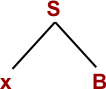Step 02: W=  xxBB                    (Using B → xBB)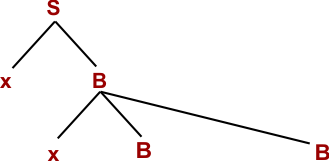Step 03: W=  xxBxBB                 (Using B → xBB)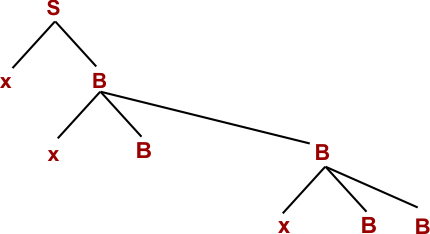Step 04: W=  xxBxByS               (Using B → yS)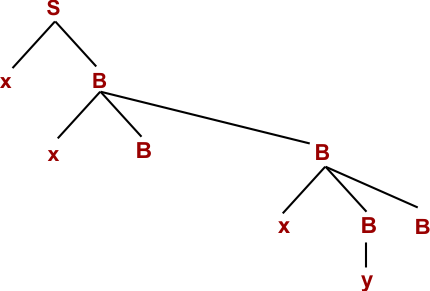Step 05: W=  xxBxByyA             (Using S → yA)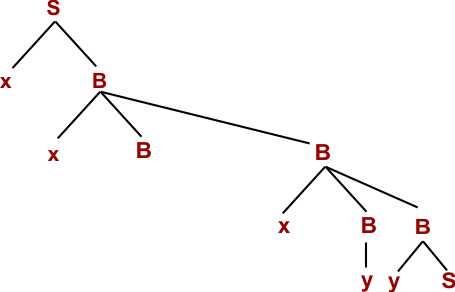Step 06: W= xxBxByyx              (Using A → x)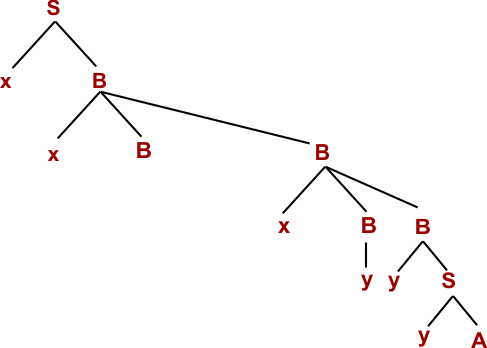Step 07: W= xxBxyyyx              (Using B → y)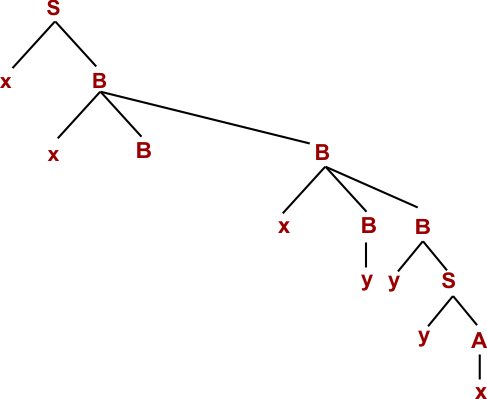Step 08: W=  xxxBBxyyyx          (Using B → xBB)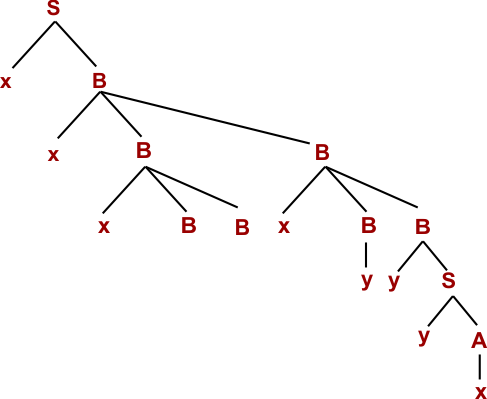Step 09: W=  xxxByxyyyx          (Using B → y)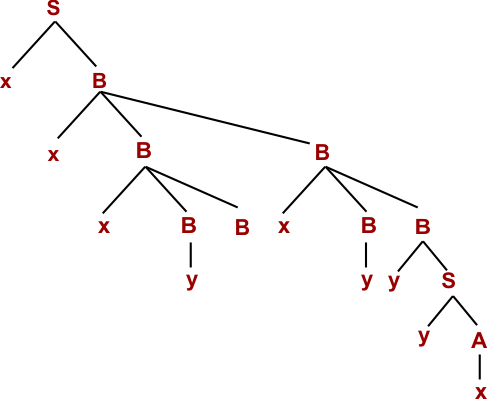Step 10: W= xxxyyxyyyx           (Using B → y)Hence proved the given String (W=xxxyyxyyyx) is derived through Rightmost derivation. So, given string belongs to Given Grammar (G).

Help Other’s By Sharing…